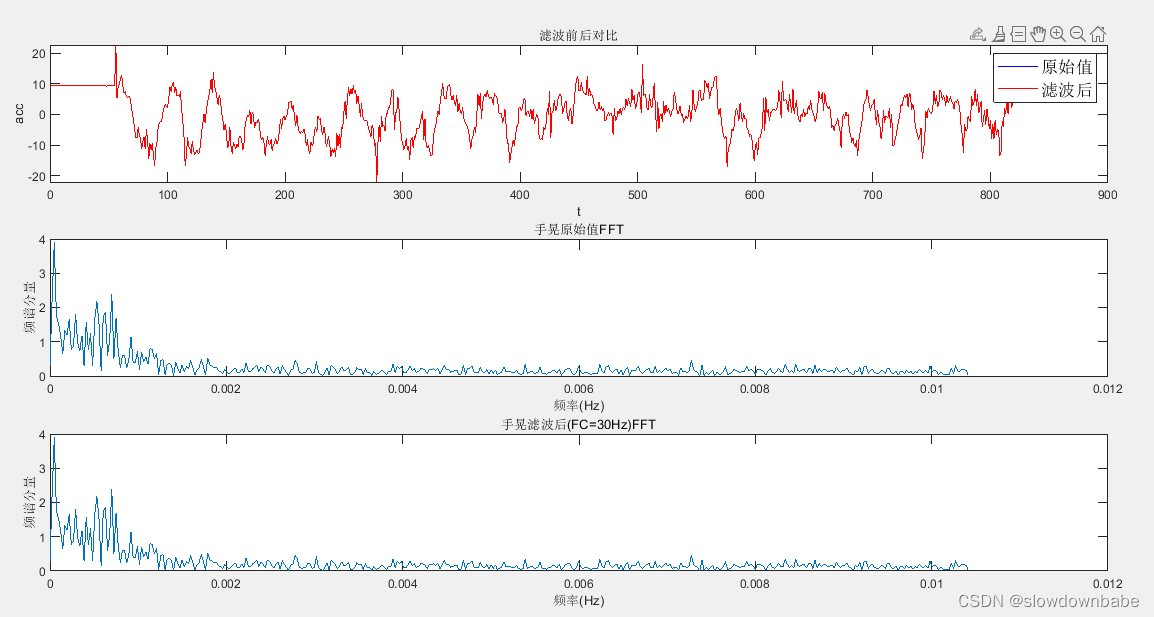# 实现STM32低通滤波器的代码

## 一、一阶低通滤波器

1. 公式

`````` lpfdata += (1.0 / (1.0 + 1.0/(2.0f * 3.14f *T*fc)))*(rawdata - lpfdata );
``````

lpfdata ： 滤波后的数据。
rawdata ： 滤波前的原始数据。
T： 数据的采样频率的倒数，即采样周期，单位是秒。
fc : 截止频率。截止频率就是超过该频率的数据（噪声）都被过滤掉，只保留低于该截止频率的数据。

1. 原理说明参考该文章

`````` https://zhuanlan.zhihu.com/p/41328501
``````

## 二、二阶IIR低通滤波器

1. 公式

`````` 差分公式 y(n) = b0*x(n) + b1*x(n-1) + b2*x(n-2) - a1*y(n-1) - a2*y(n-2);
但是一般不用上面的公式，而是使用下面的共用延时模块的公式
d(n) = x(n) -a1*d(n-1) - a2*d(n-2);
y(n) = b0*d(n) + b1*d(n-1) + b2*d(n-2);
``````
2. 原理说明

`````` 一阶滤波那篇文章的大神还写了二阶IIR低通滤波原理。
``````

## 三 、代码

``````我的另外一篇文章《STM32CubeIDE HAL库DMA方式驱动MPU925X（2）》一节有个链接。
``````

## 四、效果（IIR滤波）

30Hz的截止频率。滤波前后没啥差别。10Hz的截止频率，滤波前后还是没啥差别。5Hz的截止频率。1Hz的截止频率。可以看出滤波效果还是不错的，就是数据失真了。